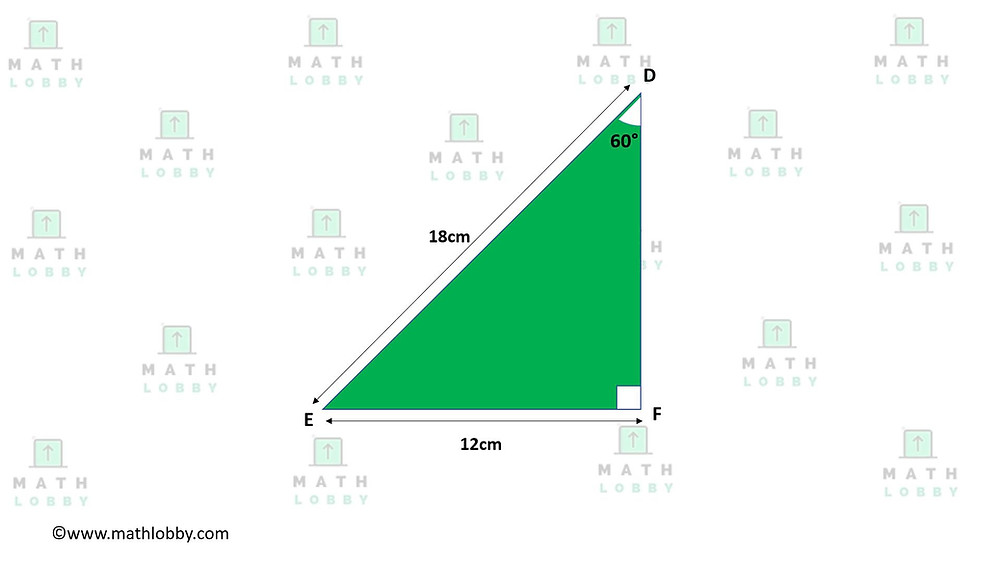Search

# Finding the area of a triangle (Using 1/2absinc)

Updated: Jun 22, 2021

Dear Secondary Math students, Math Lobby will be covering how to find the area of a triangle using the formula: ½ ab sinc. Finding the area of triangle is usually tested together with Trigonometry in O Level Math and N Level Math examinations. Make sure to check out our Trigonometry articles on our website!

In this note, you will learn:

1) How to find the area of a triangle with the formula: ½ ab sinc

2) Derivation of the formula: Area of a triangle = ½ ab sinc

### 1) How to find the area of a triangle with the formula: ½ ab sinc

Let’s say we are given a triangle ABC, and we are tasked to find the area of it.Given that AB has a length of 10cm, BC has a length of 6cm, and ABC is 60°. Find the area of the triangle.

From what we have learnt in the past, the area of a triangle has the formula: ½ x base x height

Simple! Let’s try applying that… but wait a minute, we are not provided with the height of the triangle! This is going to be a tough one, but here at Math Lobby, we never give up!

So, how can we solve this question when we are not provided with the height of the triangle?

First of all, we are provided with something else, ∠ ABC, and the length of the sides, AB and BC.

Today, we are going to introduce a very useful formula to find the area of a triangle with just two sides of a triangle and the angle between them. Let’s take a look at it:

Area of the triangle = ½ ab sinc

(where a and b are the sides of the triangle that meet to produce the angle, c.)

Now, let’s try solving the question!

Area of the triangle ABC = ½ (AB)(BC) sin(∠ ABC)

= ½ (10)(6) sin(60°)

= ½ (10)(6)(3/2)

= 25.9807621135cm2

~ 26.0cm² (corrected to 3 s.f)

And that is how you solve for the area of a triangle with the new learnt formula! Take note that this formula is applicable for all types of triangles (right-angled triangles, isosceles triangle, equilateral triangles, etc’). Hence it is extremely useful when you are provided with only limited information in a question!

### 2) Derivation of the formula: Area of a triangle = ½ ab sinc

Now that you know how to solve for the area of a triangle with the formula: ½ ab sinc, but do you know how did it come about? This might actually surprise you, but it goes back to the basic formula of finding the area of a triangle, which is ½ x base x height!

Let’s take a look at the derivation:

Given a triangle with the sides a & b, with an angle c,

We know that the formula for the area of a triangle is ½ x base x height. However, we are not provided with the height, hence what we are doing in the derived formula is essentially just trying to find the height. So, let’s begin by drawing a line in the triangle to represent the height of the triangle.From this, we can see that after drawing a vertical line to represent the height, what we produced is a smaller right-angled triangle in the big triangle. Therefore, we can apply our trigonometric functions: TOH CAH SOH.

(If you do not know what TOH CAH SOH is, you can learn it by clicking the link here!)

Since a is opposite to the right angle, it must be the hypotenuse. The height of the triangle which we have drawn is opposite to the hypotenuse, hence it must be the opposite. So, which of the trigonometric functions in “TOH CAH SOH” can we use?

That’s right! We can use the “SOH” trigonometric function which involves both the opposite and the hypotenuse! Let’s see how it done:

Sine(θ) = Opposite / Hypotenuse

Sine(c) = Height of the triangle / a

Therefore, Height of the triangle = a sinc

Now, we can substitute this back into the basic formula of a triangle,

Area of a triangle = ½ x base x height

= ½ x b x (a sinc)

= ½ ab sinc

And there you have it! This is how the formula ½ ab sinc came about!

Now, let’s try this question!Given that the triangle DEF is a ri